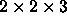# Algorithms

CS 29

Feb. 25, 1997

Working in small groups, design flowcharts and pseudo-code to solve each of the following problems. The first two problems are used within the problems following them.

1. Determine which of two numbers is larger, and print the larger number.

A simple if/else will do the trick.

2. Read numbers from a keyboard until a zero is input, then you are finished.

A simple while will do. Your algorithm doesn't have to ``do'' anything with the numbers other than read them in and compare them to zero to determine if it is finished.

3. Read positive numbers from the keyboard until the user enters a zero value, then print the largest number read.

This combines the previous two problems. The if/else will go into the body of the while loop.

Here is a hint: At the start, the largest number read is the first number read. Compare the current largest number to the number just read. The larger of them is the new current largest number. To visualize this, play a game where one person gives numbers to the second. When the first person gives a zero to the second person, the second person should say what the largest number given was.

4. Print the factors of an integer which is input from the keyboard.

For example, if the input is 42, the output should be 2 3 7.

Think about how you would factor a number like 30. Here is the general idea: see if the number is divisible by 2, then if it is divisible by 3, by 4, by 5, etc. (i.e., use a loop). Within the loop, you will need an if/else. Make sure that your solution works for numbers with repeated factors, such as 12 ().

5. Print the factors of numerous integers which are input from the keyboard. The user inputs integers one at a time. After each integer is input, you are to output the factors. If the input is zero, you are finished.

This problem makes use of the previous one and the second one. One while loop goes inside another.

Thomas P. Kelliher
Sat Feb 22 20:35:24 EST 1997
Tom Kelliher